# Monotonicity and Extremum of Functions

Monotonicity is one of the important concepts of application of derivatives. The monotonicity of a function gives an idea about the behaviour of the function. A function is said to be monotonically increasing if its graph is only increasing with increasing values of equation. Similarly, function is monotonically decreasing if its values are only decreasing.

Monotonicity: The most useful element taken into consideration amongst the total activities of the function is their monotonic behaviour. It tells about increasing or decreasing behaviour of the function.

Extremum: An extremum of a function is the point where we get the maximum or minimum value of the function in some interval.

## Monotonicity of a Function

What is monotonic function? Functions are known as monotonic if they are increasing or decreasing in their entire domain.

Examples : f(x) = 2x + 3, f(x) = log(x), f(x) = ex are the examples of increasing function and f(x) = -x5 and f(x) = e-x are the examples of decreasing function.

Increasing function: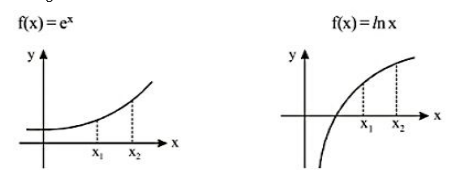If x1 < x2 and F(x1) < F(x2) then function is known as increasing function or strictly increasing function.

Decreasing function:

For F(x) = e(-x)If x1 < x2 and F(x1) > F(x2) then function is known as decreasing function or strictly decreasing function.

### Non monotonic Function

The functions which are increasing as well as decreasing in their domain are known as non monotonic function.

Example: f(x) = sin x , f(x) = |x| are examples of non monotonic function. But f(x) = sin x is increasing in [0, Π/2] or we can say it is monotonic in [0, Π/2]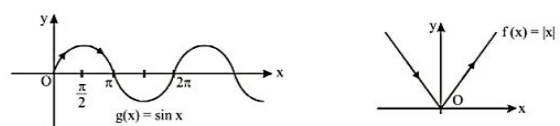## Monotonicity of a function at a point

A function is said to be monotonically increasing at x = a if it satisfies

f(a + h) > f(a)

f(a – h) < f(a)

Where h is very small value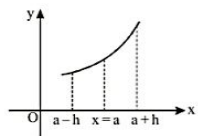A function is said to be monotonically decreasing at x = a if it satisfies

f(a + h) < f(a)

f(a – h) > f(a)

Where h is very small value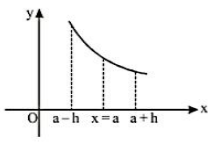Note: We can talk about the monotonicity of a function at x = a only if x = a is in the domain of the function and we don’t need to take continuity and differentiability in consideration.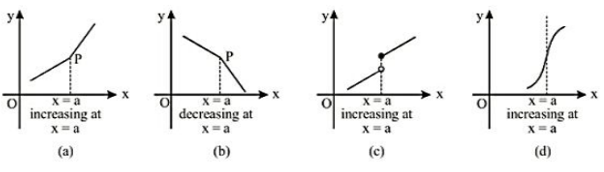## Monotonicity in an Interval

(a). For an increasing function in some interval,

If dy/dx > 0 for all the values of x belongs to that interval , then function is known as monotonically increasing or strictly increasing function.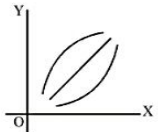(b). For an decreasing function in some interval,

If dy/dx < 0 for all the values of x belongs to that interval , then function is known as monotonically decreasing or strictly decreasing function.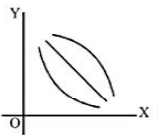Note: hence to find the interval of monotonicity for a function y = f(x) we need to find out the value of dy/dx and have to solve the inequality dy/dx > 0 0r dy/dx < 0. The solution of this inequality gives the interval of monotonicity.

Note: If dy/dx = 0 for a function y = f(x), still the function can be increasing at x = a. Consider a function f(x) = x3 which is increasing at x = 0 although dy/dx = 0. This is because f(0 + h) > f(0) and f(0 – h) < f(0).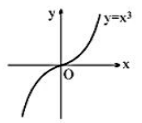All those points where dy/dx = 0 but function is still increasing or decreasing are known as Point of Inflection, which indicate the change of concavity.

## Increasing and Decreasing Function

(a). For a function y = f(x) to be monotonically increasing dy/dx ≥ 0 for all such values of interval (a,b) and equality may hold for discrete values.

(b). For a function y = f(x) to be monotonically decreasing dy/dx ≤ 0 for all such values of interval (a,b) and equality may hold for discrete values.

Note: by discrete points, we mean all those points where dy/dx = 0 does not form any interval.

## Maxima and Minima

• Local Maxima: is defined as the point on the curve where the function value at the point is greater than the limiting function value.
• Local Minima: is defined as the point on the curve where the function value at the point is lesser than the limiting function value.
• Global Maxima: is the highest value of the function among the various critical points of the function.
• Global Minima: is the least value of the function among the various critical points of the function.

## Extremum of functions

Extremum of functions is the least and the greatest values of the function. There are three different cases for all such values and those are:

Case 1 : If a function y = f(x) is the strictly increasing function in an interval [a,b], then f(a) is the least value and f(b) is the greatest value as shown in figure-1.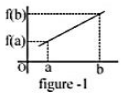Case 2 : If a function y = f(x) is the strictly decreasing function in an interval [a,b], then f(a) is the greatest value and f(b) is the lowest value as shown in figure-2.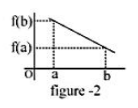Case 3 : if a function y = f(x) is non monotonic in interval [a,b] and is continuous then the greatest and the least value of the function are at those points where dy/dx = 0 or where dy/dx does not exist or at extreme values i.e. at x = a and x = b.(figure-3)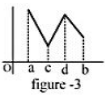## Points to remember

1] A function might consist of many local maxima and local minima but only one global maximum and one global minimum.

2] The value of the local maximum or local minimum may or may not be the global maxima or global minima.

3] The value of the local maximum may be less than a local minimum at some point.

4] For any given continuous function, the happening of minima and maxima is alternate.

## Examples on Monotonicity and Extremum of functions

Example 1: Prove that f(x) = x – sin(x) is an increasing function.

Solution: f(x) = x – sin(x)

=> dy/dx = 1 – cos(x)

dy/dx ≥ 0 as cos(x) having value in interval [-1,1] and dy/dx = 0 for the discrete values of x and do not form an interval, hence we can include this function in monotonically increasing function.

Example 2: Find the interval of monotonicity for f(x) = x/(log(x)).

Solution: f(x) = x/(log(x))

=> dy/dx = (log(x) – 1)/(log(x))^2

Sign of dy/dx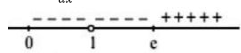So we can say that function is increasing in (e,∞) and decreasing in (0,1) U (1,e).

Example 3: Find the interval of monotonicity for f(x) = f(x) = x3/3 + (5x)2/2 + 6x.

Solution: f(x) = x3/3 + (5x)2/2 + 6x

=> f’(x) = x+ 5x + 6

For strictly increasing:

f’(x) > 0

=> x2 + 5x + 6 > 0

=> x ϵ (∞,-3) U (-2,∞)

For strictly decreasing :

f’(x) < 0

=> x2 + 5x + 6 < 0

=> x ϵ (-3,-2).

Example 4: Find the extremum of function f(x) = 3x3 – 9x in the interval [-1, 4]

Solution: f(x) = 3x3 – 9x

=> f’(x) = 9x2 – 9 = 9(x2 – 1)

=> f’(x) = 0 => 9(x– 1) = 0 or x = ±1

=> f(-1) = 6

=> f(1) = – 6

=> f(4) = 156

=> Greatest value = 156 and least value = – 6.

Example 5: In what interval is the function $\sin x-\cos x$ increasing?

Solution:

We have, $f'(x)=\cos x+\sin x$

Now, f(x) is increasing function of x, if $f'(x)=\cos x+\sin x>0$ or

$\sqrt{2}\cos \left( x-\frac{\pi }{4} \right)>0$ $0\le x<\frac{3\pi }{4}\ i.e.\,\,\,f'(x)>0$ in $\left[ 0,\frac{3\pi }{4} \right)$